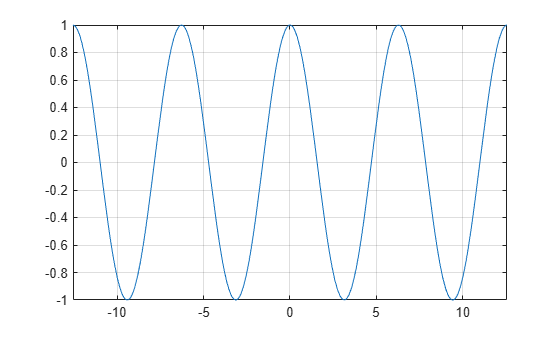# cos

Symbolic cosine function

## Syntax

``cos(X)``

## Description

example

``cos(X)` returns the cosine function of `X`.`

## Examples

### Cosine Function for Numeric and Symbolic Arguments

Depending on its arguments, `cos` returns floating-point or exact symbolic results.

Compute the cosine function for these numbers. Because these numbers are not symbolic objects, `cos` returns floating-point results.

`A = cos([-2, -pi, pi/6, 5*pi/7, 11])`
```A = -0.4161 -1.0000 0.8660 -0.6235 0.0044```

Compute the cosine function for the numbers converted to symbolic objects. For many symbolic (exact) numbers, `cos` returns unresolved symbolic calls.

`symA = cos(sym([-2, -pi, pi/6, 5*pi/7, 11]))`
```symA = [ cos(2), -1, 3^(1/2)/2, -cos((2*pi)/7), cos(11)]```

Use `vpa` to approximate symbolic results with floating-point numbers:

`vpa(symA)`
```ans = [ -0.41614683654714238699756822950076,... -1.0,... 0.86602540378443864676372317075294,... -0.62348980185873353052500488400424,... 0.0044256979880507857483550247239416]```

### Plot Cosine Function

Plot the cosine function on the interval from $-4\pi$ to $4\pi$.

```syms x fplot(cos(x),[-4*pi 4*pi]) grid on```### Handle Expressions Containing Cosine Function

Many functions, such as `diff`, `int`, `taylor`, and `rewrite`, can handle expressions containing `cos`.

Find the first and second derivatives of the cosine function:

```syms x diff(cos(x), x) diff(cos(x), x, x)```
```ans = -sin(x) ans = -cos(x)```

Find the indefinite integral of the cosine function:

`int(cos(x), x)`
```ans = sin(x)```

Find the Taylor series expansion of `cos(x)`:

`taylor(cos(x), x)`
```ans = x^4/24 - x^2/2 + 1```

Rewrite the cosine function in terms of the exponential function:

`rewrite(cos(x), 'exp')`
```ans = exp(-x*1i)/2 + exp(x*1i)/2```

### Evaluate Units with `cos` Function

`cos` numerically evaluates these units automatically: `radian`, `degree`, `arcmin`, `arcsec`, and `revolution`.

Show this behavior by finding the cosine of `x` degrees and `2` radians.

```u = symunit; syms x f = [x*u.degree 2*u.radian]; cosinf = cos(f)```
```cosinf = [ cos((pi*x)/180), cos(2)]```

You can calculate `cosinf` by substituting for `x` using `subs` and then using `double` or `vpa`.

## Input Arguments

collapse all

Input, specified as a symbolic number, scalar variable, matrix variable (since R2021a), expression, or function, or as a vector or matrix of symbolic numbers, scalar variables, expressions, or functions.

collapse all

### Cosine Function

The cosine of an angle, α, defined with reference to a right angled triangle isThe cosine of a complex argument, α, is

`$\text{cos}\left(\alpha \right)=\frac{{e}^{i\alpha }+{e}^{-i\alpha }}{2}\text{\hspace{0.17em}}.$`